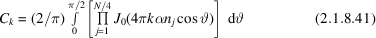International
Tables for
Crystallography
Volume B
Reciprocal space
Edited by U. Shmueli

International Tables for Crystallography (2006). Vol. B. ch. 2.1, p. 208   | 1 | 2 |

## Section 2.1.8.6. Other non-ideal Fourier p.d.f.'s

U. Shmuelia* and A. J. C. Wilsonb

aSchool of Chemistry, Tel Aviv University, Tel Aviv 69 978, Israel, and bSt John's College, Cambridge, England
Correspondence e-mail:  ushmueli@post.tau.ac.il

#### 2.1.8.6. Other non-ideal Fourier p.d.f.'s

| top | pdf |

As pointed out above, the representation of the p.d.f.'s by Fourier series is also applicable to effects of noncrystallographic symmetry. Thus, Shmueli et al. (1985) obtained the following Fourier coefficient for the bicentric distribution in the space groupto be used with equation (2.1.8.5). Furthermore, if we use the important property of the characteristic function as outlined in Section 2.1.4.1, it is easy to write down the Fourier coefficient for aasymmetric unit containing a centrosymmetric fragment centred at a noncrystallographic centre and a number of atoms not related by symmetry. This Fourier for the above partially bicentric arrangement is a product of expressions (2.1.8.17)and (2.1.8.41), with the appropriate number of atoms in each factor (Shmueli & Weiss, 1985a). While the purely bicentric p.d.f. obtained by using (2.1.8.41)with (2.1.8.5)is significantly different from the ideal bicentric p.d.f. given by equation (2.1.5.13)only when the atomic composition is sufficiently heterogeneous, the above partially bicentric p.d.f. appears to be a useful development even for an equal-atom structure.

The problem of the coexistence of several noncrystallographic centres of symmetry within the asymmetric unit of P, and its effect on the p.d.f. of, was examined by Shmueli, Weiss & Wilson (1989) by the Fourier method. The latter study indicates that the strongest effect is produced by the presence of a single noncrystallographic centre.

Another kind of noncrystallographic symmetry is that arising from the presence of centrosymmetric fragments in a noncentrosymmetric structure – the subcentric arrangement already discussed in Section 2.1.5.4. A Fourier-series representation of a non-ideal p.d.f. corresponding to this case was developed by Shmueli, Rabinovich & Weiss (1989), and was also applied to the mathematically equivalent effects of dispersion and presence of heavy scatterers in centrosymmetric special positions in a noncentrosymmetric space group.

A variety of other non-ideal p.d.f.'s occur when heavy atoms are present in special positions (Shmueli & Weiss, 1988). Without going into the details of this development, it can be noted that if the atoms are distributed among k types of Wyckoff positions, the characteristic function corresponding to the p.d.f. ofis a product of the k characteristic functions, each of which is related to one of these special positions; the same property of the characteristic function as that in Section 2.1.4.1is here utilized.

### ReferencesShmueli, U., Rabinovich, S. & Weiss, G. H. (1989). Exact conditional distribution of a three-phase invariant in the space group P1. I. Derivation and simplification of the Fourier series. Acta Cryst. A45, 361–367.Google ScholarShmueli, U. & Weiss, G. H. (1985a). Centric, bicentric and partially bicentric intensity statistics. Structure and statistics in crystallography, edited by A. J. C. Wilson, pp. 53–66. Guilderland: Adenine Press.Google ScholarShmueli, U. & Weiss, G. H. (1988). Exact random-walk models in crystallographic statistics. IV. P.d.f.'s ofallowing for atoms in special positions. Acta Cryst. A44, 413–417.Google ScholarShmueli, U., Weiss, G. H. & Kiefer, J. E. (1985). Exact random-walk models in crystallographic statistics. II. The bicentric distribution in the space group. Acta Cryst. A41, 55–59.Google ScholarShmueli, U., Weiss, G. H. & Wilson, A. J. C. (1989). Explicit Fourier representations of non-ideal hypercentric p.d.f.'s of. Acta Cryst. A45, 213–217.Google Scholar